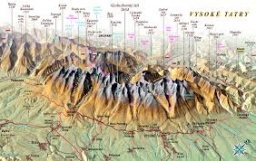# Distance 6460

Tomas will leave point A at a speed of 4 km / h at 9 o'clock. Ondra will meet him from point B at a speed of 3 km / h at 9.30 am. The distance between points A and B is 5.5km. What time do they meet, and how far will they be from point B?

Result

t = 10:00s =  1.5 km

### Step-by-step explanation:

4 · (t-9.00) + s = 5.5
s = 3·(t-(9+30/60))

s+4t = 41.5
60s-180t = -1710

Pivot: Row 1 ↔ Row 2
60s-180t = -1710
s+4t = 41.5

Row 2 - 1/60 · Row 1 → Row 2
60s-180t = -1710
7t = 70

t = 70/7 = 10
s = -1710+180t/60 = -1710+180 · 10/60 = 1.5

s = 3/2 = 1.5
t = 10

Our linear equations calculator calculates it.Did you find an error or inaccuracy? Feel free to write us. Thank you!

Tips for related online calculators
Do you have a linear equation or system of equations and looking for its solution? Or do you have a quadratic equation?
Do you want to convert velocity (speed) units?